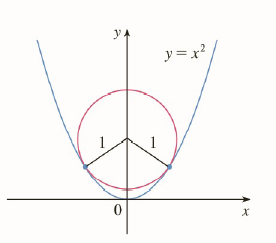Chapter 2.P, Problem 9P

Chapter
Section
Textbook Problem

# The figure shows a circle with radius 1 inscribed in the parabola y = x 2 . Find the center of the circle.To determine

To Find: The center of the circle.

Explanation

1) Concept:

Use given information and equation of circle with center (a, b) and radius r.

From the given figure circle touches parabola at two points. These points satisfy equation of the circle.

We can use this to find center of circle.

Another way to solve the problem is to notice that the at point where they touch the circle and parabola has same tangent. We can solve for c by equating the slope of the tangent.

2) Formula:

Equation of circle with center (a, b) and radius r is given by,x-a2+y-b2=r2

3) Given:

Circle with radius 1 is inscribed in parabola y=x2.

4) Calculations:

From given figure center of circle is (0, c) where c is y-intercept.

Equation of circle with center (0, c) and radius 1 is given by,

x2+y-c2=1

As parabola intersects circle at two points, therefore, equation of parabola y= x2 satisfy equation of circle.

Using y=x2 in equation of circle,

y+y-c2=1

y+y2-2cy+c2=1

y2-2c-1y+c2-1=0

Parabola intersects circle at two points but from figure y- coordinates of these points are same.

Therefore, discriminant of above polynomial is 0.

Hence 2c-12-4c2-1=0

4c2-4c+1-4c2+4=0

-4c+5=0

c=54

Alternate method:

Let (a, b) be a common point of the circle and tangent. Consider the common tangent at this point. We can find the slope of this tangent by evaluating the derivative of either of the curve at x=a. Let this slope be m.

Equation of parabola is y=x2. Differentiating it we get

dydx=2x

Thus the slope of tangent at (a, b)

m =2a

Now the line is tangent to the circle as well

### Still sussing out bartleby?

Check out a sample textbook solution.

See a sample solution

#### The Solution to Your Study Problems

Bartleby provides explanations to thousands of textbook problems written by our experts, many with advanced degrees!

Get Started

#### Find more solutions based on key concepts